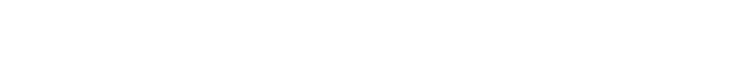# 2.2.4 Leptons

### Leptons & Antileptons

• Leptons are a group of fundamental (elementary) particles
• This means they are not made up of any other particles (no quarks)
• Leptons interact with other particles via the weak, gravitational or electromagnetic interactions
• They do not interact via the strong nuclear force
• The most common leptons are:
• The electron, e
• The electron neutrino, ve
• The muon, μ
• The muon neutrino, vμThe most common leptons are the electron, muon and their respective neutrinos

• The muon is similar to the electron but is slightly heavier
• Electrons and muons both have a charge of -1e and a mass of 0.0005u
• Neutrinos are the most abundant leptons in the universe and have no charge and negligible mass (almost 0)
• Although quarks are fundamental particles too, they are not classed as leptons
• Leptons do not interact with the strong force, whilst quarks do

#### Worked Example

Circle all the anti-leptons in the following decay equation.### Lepton Number

• Similar to baryon number, the lepton number, L is the number of leptons in an interaction
• L depends on whether the particle is a lepton, anti-lepton or neither
• Leptons have a lepton number L = +1
• Anti-leptons have a lepton number L = –1
• Particles that are not leptons have a lepton number L = 0
• Lepton number is a quantum number and is conserved in all interactions
• This is helpful for knowing whether an interaction is able to happenThe lepton number depends if the particle is a lepton, anti-lepton or neither

#### Worked Example

If the lepton number is conserved in the following decay, identify whether particle X should be a neutrino or anti-neutrinoStep 1: Determine the lepton number of all the particles on both sides of the equation

0 + (–1) = 0 + X

Step 2: Identify the lepton number of X

If the lepton number must be conserved, X must also have a lepton number of –1

Step 3: State the particle X

Particle X is an anti-neutrino

#### Exam Tip

No need to fret about memorising all the different lepton numbers – the lepton number of each lepton and anti–lepton is provided on the datasheet.

Remember that although quarks are fundamental particles, they have a lepton number of 0 and are classed as baryons.

### Muon Decay

• Muons are leptons that are slightly heavier than the electron
• Muons (μ) typically decay into an electron
• Anti-muons (μ+) typically decay into positrons• Muon decay occurs through the weak interaction
• This can be recognised by the exchange of the W boson on a Feynman diagram### Author: Ashika

Ashika graduated with a first-class Physics degree from Manchester University and, having worked as a software engineer, focused on Physics education, creating engaging content to help students across all levels. Now an experienced GCSE and A Level Physics and Maths tutor, Ashika helps to grow and improve our Physics resources.
CloseClose